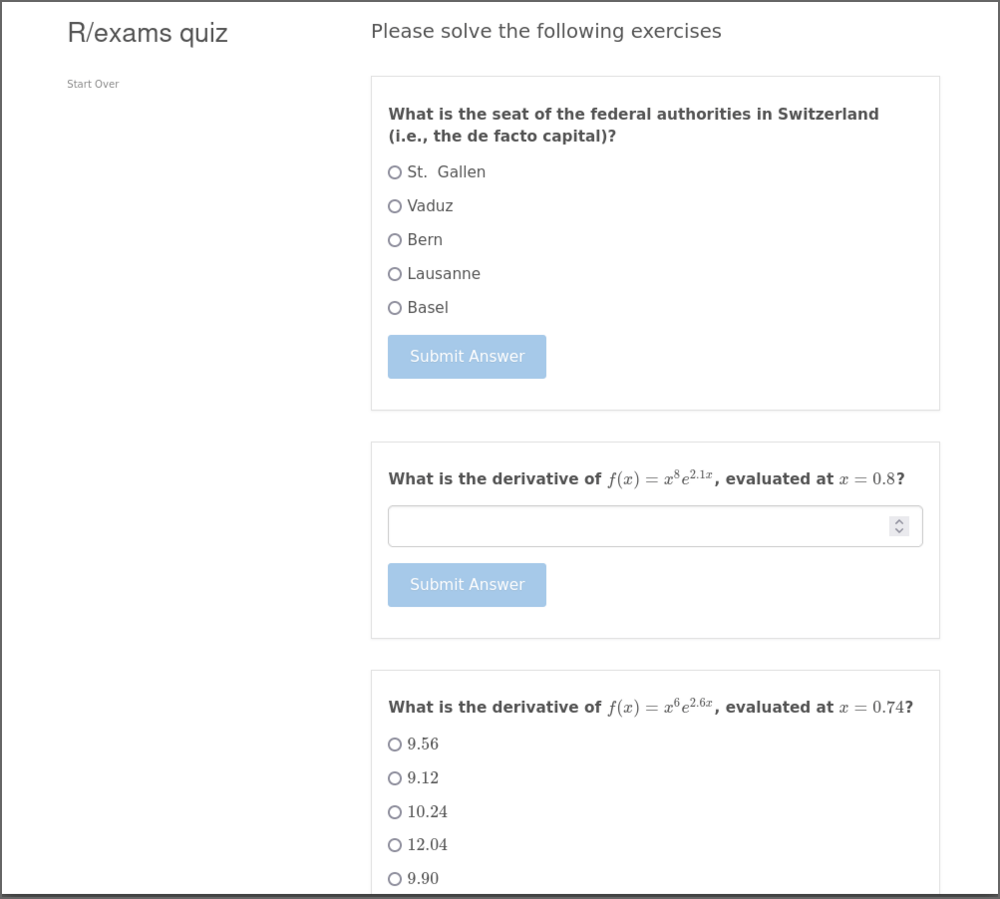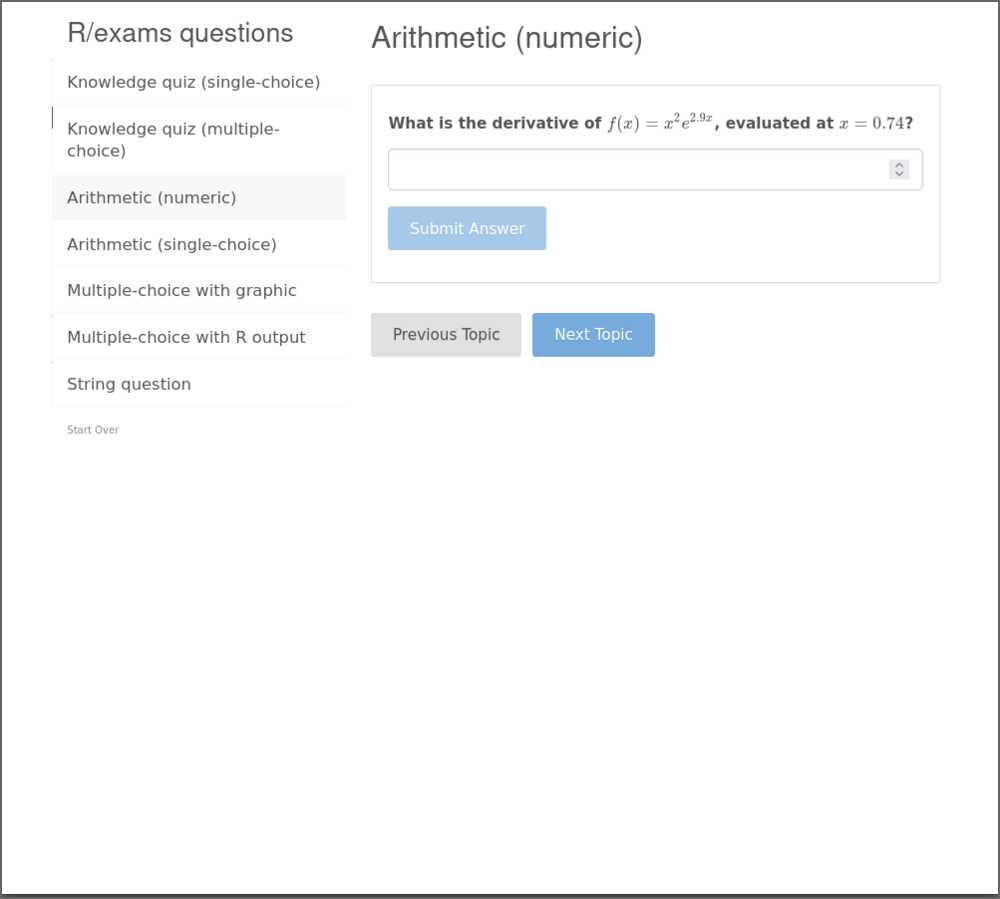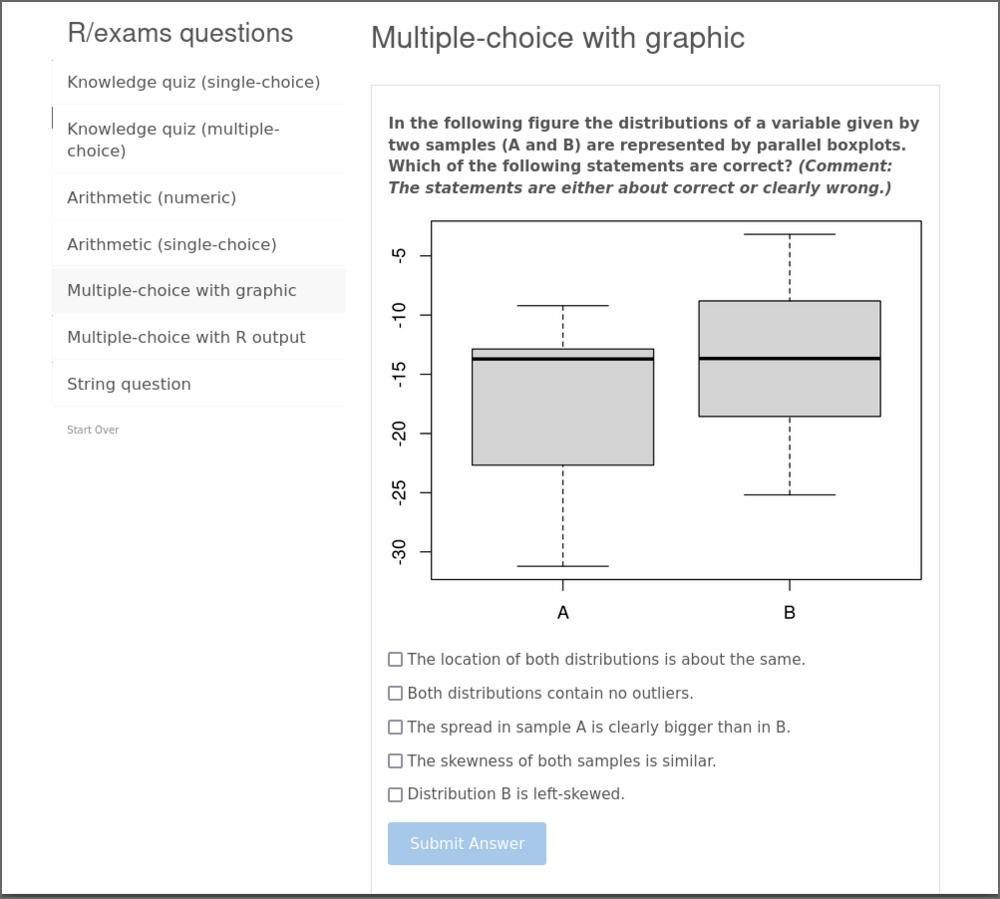Want to share your content on R-bloggers? click here if you have a blog, or here if you don't.

Introduction to the new exams2learnr package for including quizzes or individual questions from dynamic exercise templates into learnr tutorials which can be deployed as shiny apps.## Overview

The package `exams2learnr` mainly provides the function of the same name, `exams2learnr()`. It is an interface for embedding exercises written with R/exams in tutorials or quizzes written with the learnr package.

The goal of `learnr` is the creation of interactive tutorials in a shiny application written with R/Markdown. The tutorials can contain individual questions (exercises) or quizzes (sets of exercises) along with text, graphics, and other elements you can embed in R/Markdown. Usually, these tutorials can then be used for self-paced learning rather than (summative) assessment.

The `exams2learnr()` interface leverages `learnr`’s capabilities for questions/quizzes to dynamically insert (random variations) from R/exams exercise templates, either written in Rmd (R/Markdown) or Rnw (R/LaTeX) format. Currently, there is support for the following question types:

Description R/exams learnr
Multiple-choice mchoice learnr_checkbox
Numeric num learnr_numeric
Text string learnr_text

Thus, there is no support, yet, for `cloze` questions that can combine all of the elements above.

## First motivation

For quickly trying out how a certain R/exams exercise is rendered in a `learnr` tutorial, there is the convenience function `run_quiz()`. This sets up a tutorial embedding R/exams exercises in a temporary directory and directly runs the tutorial in a `shiny` app. For illustration, the code below creates a quiz containing one random version of each of these exercises that are shipped with the R/exams package: capitals (multiple-choice), fruit (numeric), function (string/text). A screenshot is included below the code.

```library("exams2learnr")
run_quiz(c("capitals.Rmd", "fruit.Rmd", "function.Rmd"))
```Thus, the `run_quiz()` function is nice for getting a first feeling for what is possible with the `exams2learnr` package and for the look & feel of the resulting `shiny` apps. For full customization, however, a dedicated Rmd tutorial should be set up. This can then leverage the main `exams2learnr()` function to explicitly include questions/quizzes, potentially along with further elements and/or customizations.

## Building blocks

The main function `exams2learnr()` can take one or more exercise templates, either as a vector or as a list, just as for other `exams2xyz()` interfaces. By default, this creates a single random replication from (each of) the exercise(s), converts the text to HTML, and wraps it into a suitable `learnr` object. For a single exercise a `tutorial_question` is returned and for a vector/list of exercises a `tutorial_quiz`. These defaults can be modified and further arguments can be passed on to the underlying `learnr` function calls to `question()` and `quiz()`, respectively. This allows for customizing the appearance or controlling whether multiple attempts are allowed, or whether hints/solutions are shown, etc.

As a first example, we apply `exams2learnr()` to the string/text function exercise, readily provided within the R/exams package. The result is represented as a `learnr_text` object, inheriting from `tutorial_question`:

```qn <- exams2learnr("function.Rmd")
class(qn)
##  "learnr_text"       "tutorial_question"
print(qn)
## Question: "What is the name of the R function for extracting the fitted
## log-likelihood from a fitted (generalized) linear model object?"
##   type: "learnr_text"
##   allow_retry: FALSE
##     ✔: "logLik"
##   messages:
##     correct: "Correct!"
##     incorrect: "Incorrect"
##     message: "<code>logLik</code> is the R function for extracting the
##     fitted log-likelihood from a fitted (generalized) linear model object.
## See <code>?logLik</code> for the corresponding manual page."
##   Options:
##     trim: TRUE
```

Subsequently, we set up a quiz with the single-choice swisscapital exercise and the multiple-choice switzerland exercise, represented as `learnr_radio` and `learnr_checkbox` objects, respectively, contained in a `tutorial_quiz`.

```qz <- exams2learnr(c("swisscapital.Rmd", "switzerland.Rmd"))
class(qz)
##  "tutorial_quiz"
print(qz)
## Quiz: "Quiz"
##
##   Question: "What is the seat of the federal authorities in Switzerland
##   (i.e., the de facto capital)?"
##     allow_retry: FALSE
##       X: "Zurich"
##       X: "Basel"
##       ✔: "Bern"
##       X: "Geneva"
##     messages:
##       correct: "Correct!"
##       incorrect: "Incorrect"
##       message: "There is no de jure capital but the de facto capital and
##       seat of the federal authorities is Bern.</p>
##
## <ul>
## <li>False</li>
## <li>False</li>
## <li>True</li>
## <li>False</li>
## <li>False</li>
## </ul>
## "
##
##   Question: "Which of the following statements about Switzerland is correct?"
##     type: "learnr_checkbox"
##     allow_retry: FALSE
##       X: "The currency in Switzerland is the Euro."
##       ✔: "Italian is an official language in Switzerland."
##       ✔: "The Swiss national holiday is August 1."
##       X: "Switzerland is part of the European Union (EU)."
##       X: "Zurich is the capital of Switzerland."
##     messages:
##       correct: "Correct!"
##       incorrect: "Incorrect"
##       message: "<ul>
## <li>False. The currency is the Swiss Franc (CHF).</li>
## <li>True. The official languages are: German, French, Italian, Romansh.</li>
## <li>True. The establishment of the Swiss Confederation is traditionally dated to August 1, 1291.</li>
## <li>False. Switzerland is part of the Schengen Area but not the EU.</li>
## <li>False. There is no de jure capital but the de facto capital of Switzerland is Bern.</li>
## </ul>
## "
```

If more than `n = 1` random replications are produced then a standard list is returned – because `learnr` currently does not provide a compound object that can capture a question bank with multiple replications. The list has one element per random replication, each of which is a list of `tutorial_question` objects.

The format of the return value can also be controlled by the `output` argument if the desired format is not selected automatically.

## Tutorial with a quiz

For setting up a `learnr` tutorial with a quiz based on R/exams exercises the building blocks discussed above can be used. In the YAML header the `output` should be `learnr::tutorial` and the `runtime` should to be `shiny_prerendered`. Then the quiz (or “exam”) can be set up as a list of exercise templates, as for other `exams2xyz()` interfaces as well. The entire quiz is then prepared in a single `exams2learnr()` call. For illustration consider:

```---
title: "R/exams quiz"
output: learnr::tutorial
runtime: shiny_prerendered
---

```{r setup, include = FALSE}
## package and list of various exercises
library("exams2learnr")
exm <- list(
c("swisscapital.Rmd", "capitals.Rmd"),
"deriv.Rnw",
"deriv2.Rnw",
"fruit.Rmd",
"boxplots.Rmd",
"ttest.Rmd",
"function.Rmd"
)
```

```{r rexams_quiz, echo = FALSE, message = FALSE}
exams2learnr(exm, caption = "Please solve the following exercises")
```
```

The demo file above is actually shipped as a supplementary file learnr_quiz.Rmd within the `exams2learnr` package. Hence, it can be `run()` as in the following command. The screenshot below shows (one random replication of) the first exercises. The concrete exercises will differ in every new `run()` of the tutorial.

```rmarkdown::run(system.file("learnr", "learnr_quiz.Rmd", package = "exams2learnr"))
```## Tutorial with individual questions

To finetune the appearance of the R/exams exercises within the `learnr` tutorial, e.g., for putting different exercises into different sections, it may be necessary to include each question/exercise individually (rather than an entire quiz). For illustration consider the following Rmd document that is provided as supplementary file learnr_questions.Rmd:

```---
title: "R/exams questions"
output: learnr::tutorial
runtime: shiny_prerendered
---

```{r setup, include = FALSE}
library("exams2learnr")
```

## Knowledge quiz (single-choice)

```{r schoice1, echo = FALSE, message = FALSE}
exams2learnr("swisscapital.Rmd", allow_retry = TRUE, incorrect = "Incorrect, try again.")
```

## Knowledge quiz (multiple-choice)

```{r mchoice2, echo = FALSE, message = FALSE}
exams2learnr("capitals.Rmd")
```

## Arithmetic (numeric)

```{r num3, echo = FALSE, message = FALSE}
exams2learnr("deriv.Rnw", allow_retry = TRUE)
```

## Arithmetic (single-choice)

```{r schoice4, echo = FALSE, message = FALSE}
exams2learnr("deriv2.Rnw")
```

## Multiple-choice with graphic

```{r mchoice5, echo = FALSE, message = FALSE}
exams2learnr("boxplots.Rmd")
```

## Multiple-choice with R output

```{r mchoice6, echo = FALSE, message = FALSE}
exams2learnr("ttest.Rmd")
```

## String question

```{r mchoice7, echo = FALSE, message = FALSE}
exams2learnr("function.Rmd", allow_retry = TRUE)
```
```

This tutorial also shows how to use some of `learnr`’s customization options such as `allow_retry` or `incorrect`. To `run()` this tutorial the command below can be used. As in the previous section, the concrete exercise variations will differ in every new `run()` of the tutorial.

```rmarkdown::run(system.file("learnr", "learnr_questions.Rmd", package = "exams2learnr"))
```